# Quick Please! A buffer solution composed of butyric acid and sodium butyrate has a pH of...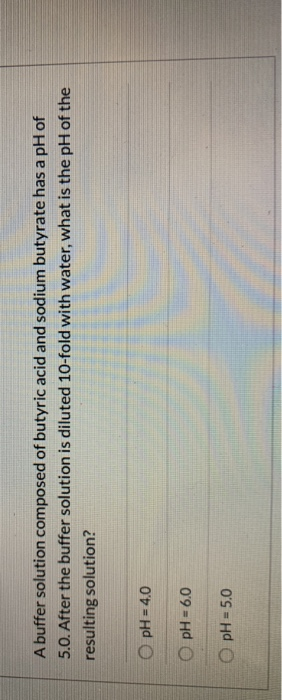A buffer solution composed of butyric acid and sodium butyrate has a pH of 5.0. After the buffer solution is diluted 10-fold with water, what is the pH of the resulting solution? O pH = 4.0 pH = 6.0 pH = 5.0

Let initial volume is 1 liter.

pH = 5

So, [H+] = 10^(-pH) = 10^(-5) = 10^-5 M

After adding water 10 folded, volume = 1*10 = 10 liters

So, new [H+] = moles/new volume = 10^-5 / 10 = 10^-6 M

So, new pH = - log [H+] = - log [10^-6] = 6

#### Earn Coin

Coins can be redeemed for fabulous gifts.

Similar Homework Help Questions
• ### A buffer made from potassium butyrate (KC4H702) and butyric acid (HC4H702) has a pH of 6.63. What is the ratio of bu...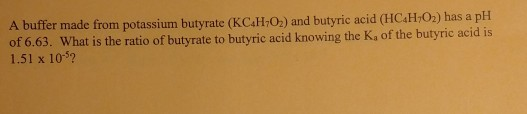A buffer made from potassium butyrate (KC4H702) and butyric acid (HC4H702) has a pH of 6.63. What is the ratio of butyrate to butyric acid knowing the K, of the butyric acid is 1.51 x 10%?

• ### Quick Please! What is the pH of a solution produced by mixing 500.0 mL of 0.200...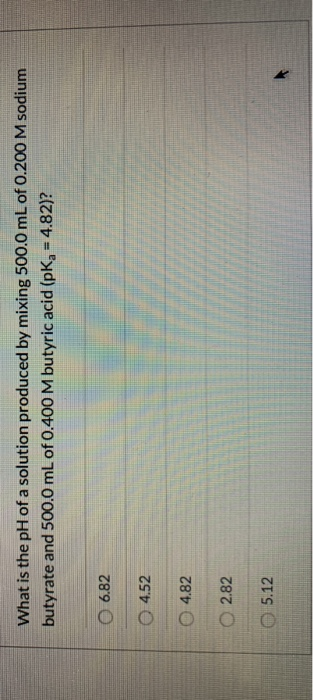Quick Please! What is the pH of a solution produced by mixing 500.0 mL of 0.200 M sodium butyrate and 500.0 mL of 0.400 M butyric acid (pK-4.82)? 0 6.82 0 4.52 0 4.82 0 2.82 05.12

• ### calculate the molar solubility of Cr(OH)3 in a solution with a pH of 11.5 knowing that...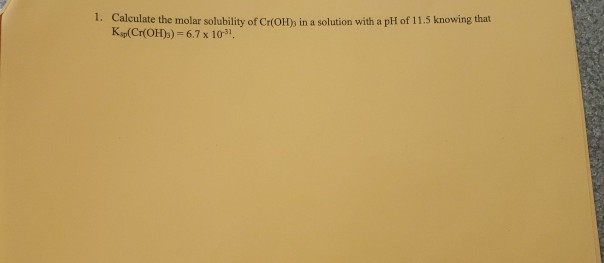calculate the molar solubility of Cr(OH)3 in a solution with a pH of 11.5 knowing that Ksp(Cr(OH)3) = 6.7 x 10^(-31) 1. Calculate the molar solubility of Cr(OH), in a solution with a pH of 11.5 knowing that Kop(Cr(OH)2) = 6.7 x 10" 2. What is the pH of a 0.895M sodium fluoride (NaF) solution knowing that the hydrofluoric acid is 7.11 x 10 of 3. Calculate the molar solubility of antimony sulfide (Sb2S) in water knowing K =1.6 x...

• ### Acid buffer described in Problem 21, you discover that your m ion? laboratory is out of sodium ac...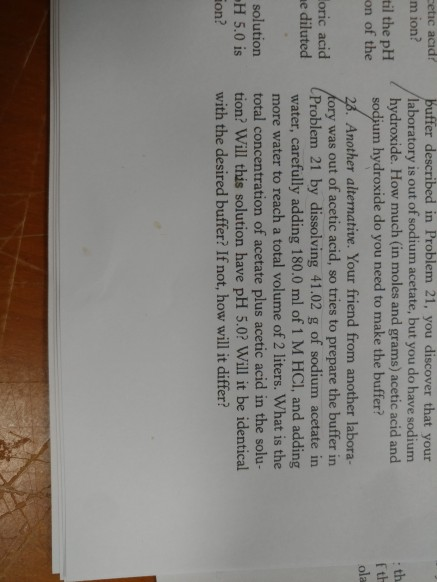number 23 acid buffer described in Problem 21, you discover that your m ion? laboratory is out of sodium acetate, but you do have sodium hydroxide. How much (in moles and grams) acetic acid and til the pH on of the sodium hydroxide do you need to make the buffer? f th ola . Another alternative. Your friend from another labora- ory was out of acetic acid, so tries to prepare the buffer in Problem 21 by dissolving 41.02 g...

• ### A 1.00 L buffer solution with pH = 4.74 is composed of 0.30 mol acetic acid...

A 1.00 L buffer solution with pH = 4.74 is composed of 0.30 mol acetic acid and 0.30 mol sodium acetate. A) Determine the pKa of acetic acid B) If 0.030 mol of NaOH is added, determine the pH of the solution

• ### A buffer solution contains .267 m acetic acid and .295 m sodium acetate. Calculate the ph...

A buffer solution contains .267 m acetic acid and .295 m sodium acetate. Calculate the ph of this buffer. If .225 moles of HBr are added to 1 L of the buffer, what is the pH of the resulting solution, assuming volume does not change? please show work and post the right answer

• ### a buffer solution of pH =5.30 can be prepared by dissolving acetic acid and sodium acetate...

a buffer solution of pH =5.30 can be prepared by dissolving acetic acid and sodium acetate in water. How many moles of sodium acetate must be added to 1 L of 0.25 M acetic acid to prepare the buffer? Ka(CH3COOH)=1.8 x 10^-5

• ### A buffer is composed of formic acid and its conjugate base, the formate ion. K for...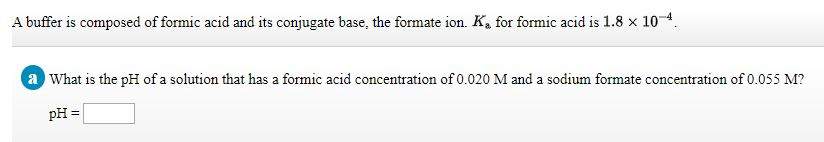A buffer is composed of formic acid and its conjugate base, the formate ion. K for formic acid is 1.8 x 10. a What is the pH of a solution that has a formic acid concentration of 0.020 M and a sodium formate concentration of 0.055 M? pH

• ### pH of a buffer solution pH and Buffers IV pH of a Buffer Solution A.2019 Mass of sodium acetate, CH,COONa, g Mea...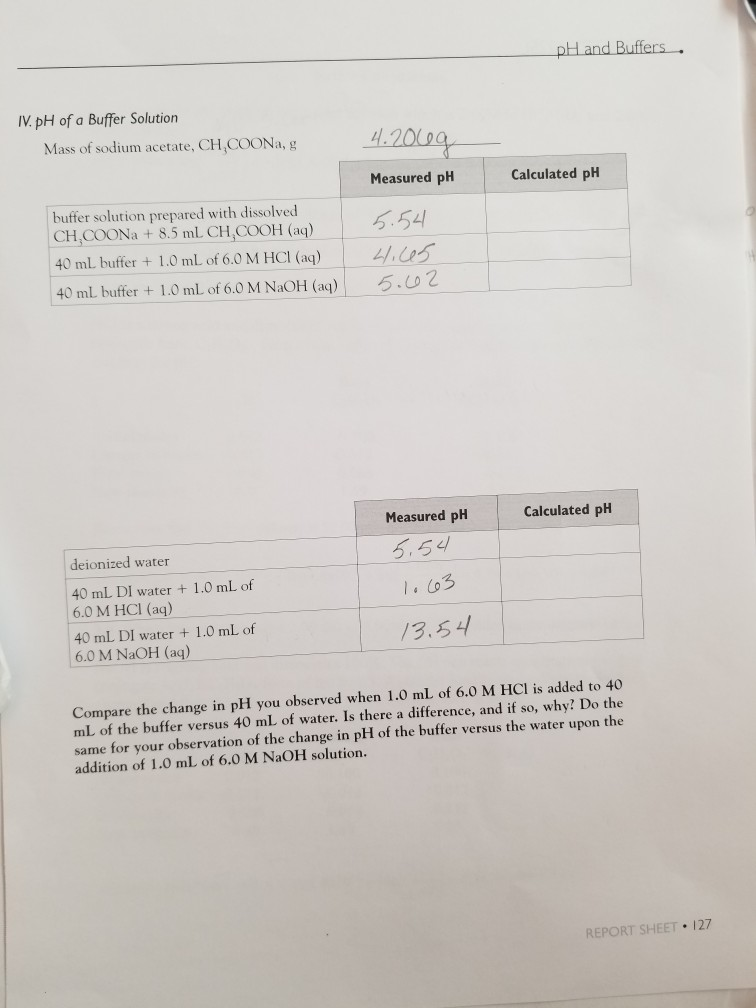pH of a buffer solution pH and Buffers IV pH of a Buffer Solution A.2019 Mass of sodium acetate, CH,COONa, g Measured pH Calculated pH buffer solution prepared with dissolved CH,COONa +8.5 mL CH,COOH (aq) 5.54 40 mL buffer + 1.0 mL of 6.0 M HCI (aq) 4/.ces 40 mL buffer + 1.0 mL of 6.0 M NaOH (aq) 5.02 Calculated pH Measured pH 5,54 deionized water 40 mL DI water +1.0 mL of 6.0 M HCI (aq) 1.C03 40...

• ### 1 . If a buffer solution is 0.260 M in a weak acid (?a=8.3×10−5)and 0.480 M in its conjugate base, what is the pH? pH= 2...

1 . If a buffer solution is 0.260 M in a weak acid (?a=8.3×10−5)and 0.480 M in its conjugate base, what is the pH? pH= 2. If a buffer solution is 0.200 M in a weak base (?b=5.0×10−5) and 0.530 M in its conjugate acid, what is the ph 3. Phosphoric acid is a triprotic acid (?a1=6.9×10−3, ?a2=6.2×10−8 , and ?a3=4.8×10−13 To find the pH of a buffer composed of H2PO4 - (aq) ) and HPO4 2− (aq) , which...